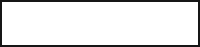##### GED Test 2022/2023 For Dummies with Online PracticeWhen you run into a three-dimensional figure problem on the GED Math test, it probably means you'll have to calculate an object's surface area or volume. Fortunately, you won't have to memorize all the different geometric formulas—they'll be supplied in a handy drop-down window—but you will have to know how to use them.

The following practice questions start by throwing you in the deep end, and asking you to find the average depth of a swimming pool; then you take on the much easier (and tastier) job of figuring out the volume of an ice cream cone.

## Practice questions

1. Igor is in charge of the swimming pool at the local recreation center. The pool is 120 feet long and 24 feet wide and holds 12,902 cubic feet of water. The average depth of the pool in feet is2. Sam and Arnold were eating ice-cream cones. Arnold wondered what volume of ice cream his cone would hold if filled to the top. Sam measured the cone and found it to be 2 1/2 inches across the top and 5 1/2 inches high. The cone would holdcubic inches of ice cream, rounded to one decimal place, if it were filled to the top.

1. The correct answer is 4.48.

This problem tests your ability to do calculations and use a formula:

2. The correct answer is 9.0.

The shape of an ice-cream cone is a cone, and the volume of a cone is

where pi is approximately equal to 3.14 (this equation appears on the formula sheet).

To make the calculations simpler using the calculator, change the fractions to decimals:

Now you have to insert the values from the question into the equation (remember that you find the radius by dividing the diameter by 2):

or 9.0 cubic inches, rounded to one decimal place.